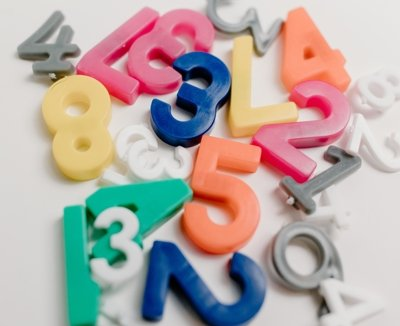The decimal to octal conversion involves dividing the decimal number by 8 successively, while storing the remainders of the divisions in reverse order. The resulting sequence of remainders is the octal number equivalent.

Decimal to octal conversion is a mathematical process that involves dividing the decimal number by 8 successively until the quotient becomes zero, while storing the remainders of the divisions in reverse order. The resulting sequence of remainders is the octal number equivalent to the original decimal number.

1. Divide the decimal number by 8.
2. Write down the remainder.
3. Divide the quotient obtained in step 1 by 8.
4. Write down the remainder.
5. Repeat steps 3 and 4 until the quotient becomes zero.
6. The resulting sequence of remainders represents the octal number equivalent of the original decimal number.

For example, to convert the decimal number 123 to octal:

1. 123 ÷ 8 = 15 with a remainder of 3. Write down 3.
2. 15 ÷ 8 = 1 with a remainder of 7. Write down 7 after the 3. We now have “73”.
3. 1 ÷ 8 = 0 with a remainder of 1. Write down 1 after the 7. We now have “173”.
4. The resulting octal number equivalent of 123 is “173”.

Example in Javascript code:

``````function decimalToOctal(decimalNumber) {
let octalNumber = "";
while (decimalNumber > 0) {
let remainder = decimalNumber % 8;
octalNumber = remainder + octalNumber;
decimalNumber = Math.floor(decimalNumber / 8);
}

return octalNumber;
}``````

Exemple in C# code:

``````public static string DecimalToOctal(int decimalNumber)
{
int quotient = decimalNumber;
string octalNumber = "";
while (quotient > 0)
{
int remainder = quotient % 8;
octalNumber = remainder.ToString() + octalNumber;
quotient /= 8;
}
return octalNumber;
}
``````

Categories:

Tags:

## Comentários

Nenhum comentário para mostrar.